# 11-Plus Exam Illustrations - Maths Quiz - Multiplication and Division of Decimals (Questions)

## Multiplication of Decimals

Multiplying a figure by a decimal is fairly straightforward and follows the same pattern as a long multiplication sum. One method is to multiply any decimals by ten, a hundred or whatever is necessary to make them into whole numbers then divide the result by the same figure. However, we will look at what I prefer to do as it is slightly simpler: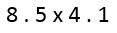The long multiplication sum is made using the digits without the decimal points. The decimal point is very important but will come back in later on, once the digits of the answer are in place. Encourage your child to make an estimate of the answer at this stage; 8 x 4 = 32 so that will do as a good reference point.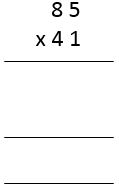Going back to the long multiplication method, we times 85 by 1 (85) and put the answer in the first row of the intermediate answer. Secondly, insert a zero in the rightmost column of the second row and work out 4 x 5 (20, so write in 0 and carry the 2) and 4 x 8 (32, add the 2 that we carried over, = 34)

Add the resulting answers together and here is the trick. How many digits were there after the decimal points in the figures we multiplied? The answer is two, 8. 5 and 4. 1, so that is how many digits will be after the decimal point in the answer.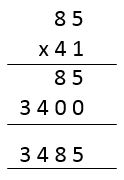The answer of 3485 will become 34.85 and of course, this fits in with the estimate of 'about 32'.

## Division of Decimals

This is a fairly intimidating thing to do without practice but you are unlikely to get a very hard question at this level. The key is to make the numbers whole and carry out long or short division and whatever the answer is, it will be the same as when the decimal points are in.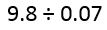We would normally look for an approximate answer but that's asking a lot for a 10-year-old. Instead, move straight to the calculation.

If we multiply the numbers by 100 then we get whole numbers. Following the technique described previously, we make 980 and 7. As we have done the same thing to both sides of the question then the division sum will give exactly the same answer to the sum 9.8 ÷ 0.07.

Setting it out as a short division sum, we get: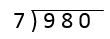Carrying this through, we get the following: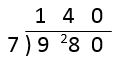Don't forget that zero, when divided by any other number, will always be zero. Similarly, any number divided by one will equal that number. E.g. 44 ÷ 1 = 44 and 0 ÷ 14 = 0. It is impossible to divide any number by zero.

The answer we made for our whole number sum is exactly the same as the answer to the decimal sum. The answer is 140.